Courses

# Bar Graphs - Introduction and Examples (with Solutions), Data Interpretation LR Notes | EduRev

## Banking Exams: Bar Graphs - Introduction and Examples (with Solutions), Data Interpretation LR Notes | EduRev

The document Bar Graphs - Introduction and Examples (with Solutions), Data Interpretation LR Notes | EduRev is a part of the Banking Exams Course General Aptitude for GATE.
All you need of Banking Exams at this link: Banking Exams

What are Bar Charts?

Bar charts are one of the easiest, graphically attractive and hence most commonly used methods of presenting all types of data. They are especially useful for representing various data series.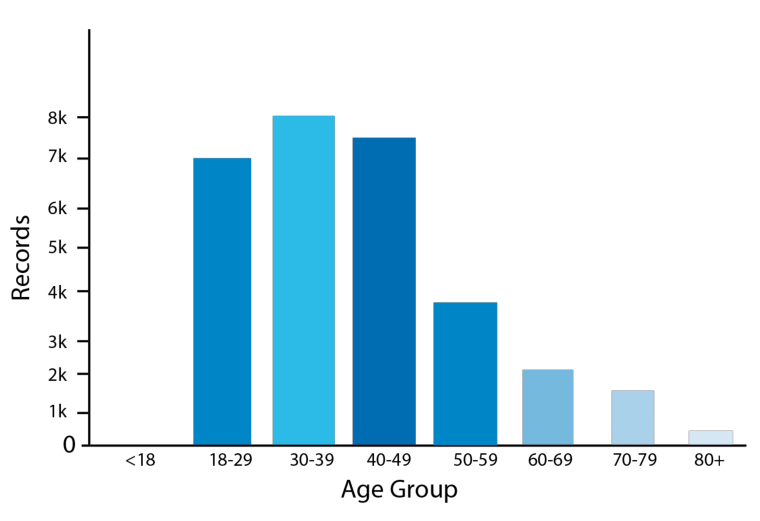Bar Chart

• The data series comprises the continuous variables while the values of the specific instances at which the value of the data series is measured represent the values of the discrete variables.
• Presentation of data as bar charts makes the comparative study of the data very easy. A bar chart consists of a group of bars that are equidistant from each other. The values on the bar charts are read by the measurement of the length or the height of the bars.
• The width of the bars is largely inessential and is used only for the clarity of the presentation.

Now let's have a look at the different kinds of bar charts and the kinds of data that can be represented on a bar chart.

1. Simple Bar Chart

The simple bar chart is the simplest bar chart that has one continuous variable charted along with one discrete variable.

Figure below shows an example of a Simple Bar Chart.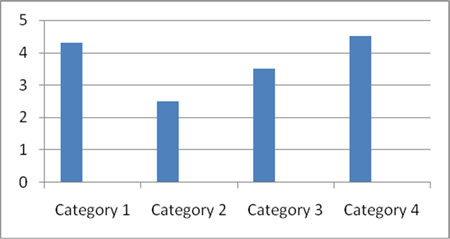Simple Bar Chart

2. Composite Bar Chart

One of the primary limitations of the simple bar chart is that it can only be used to display a single continuous variable.

• If two or more sets of continuous variables are to be shown on the same bar chart, we use what is called a composite bar chart.
• Figure below shows an example of the Composite Bar Chart.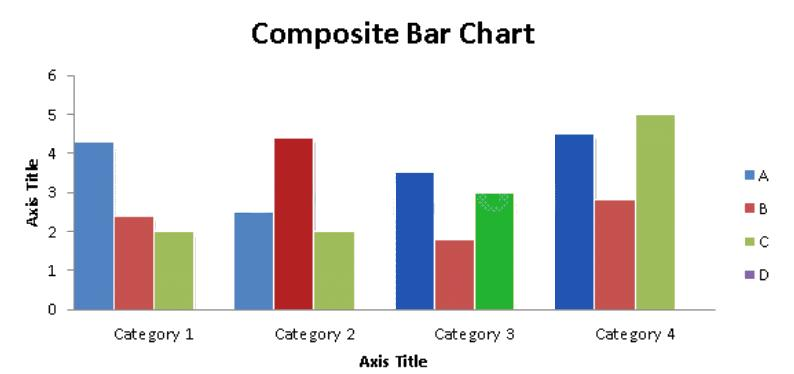Try yourself:The bar graph given below shows the sales of books (in thousand number) from six branches of a publishing company during two consecutive years 2000 and 2001.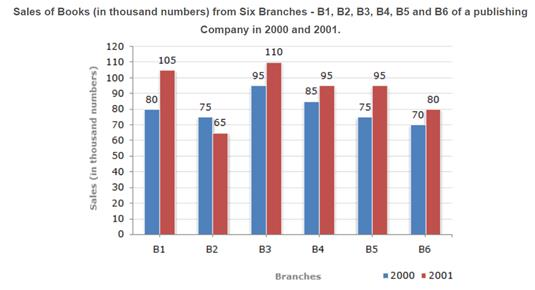What is the ratio of the total sales of branch B2 for both years to the total sales of branch B4 for both years?

The Use of Bar Charts to Show Deviations

Deviation bars are useful for graphic presentation of continuous variables which can have both positive and negative values, i.e., surplus or deficit, net profit or loss, net of imports and exports.

In general continuous variables which have both positive and negative values are best represented on bar charts.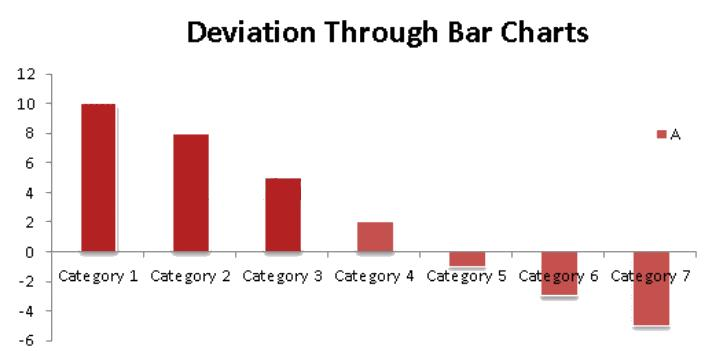• A baseline is created and positive values (such as profit, surplus), etc., are represented by hers above the baseline while negative deviations (loss or deficit) are represented by bars below the baseline as shown in the figure above.

Representation of Percentage on a Stacked Bar Chart

Sometimes stacked bars can also be used to represent the break-up of some continuous variable. The figure below will make it clear.

• Such use of bar charts is quite convenient for comparing two or more sets of data.
• The figure below shows the break-up of the various sources of revenues for the Government of India over a two-year period.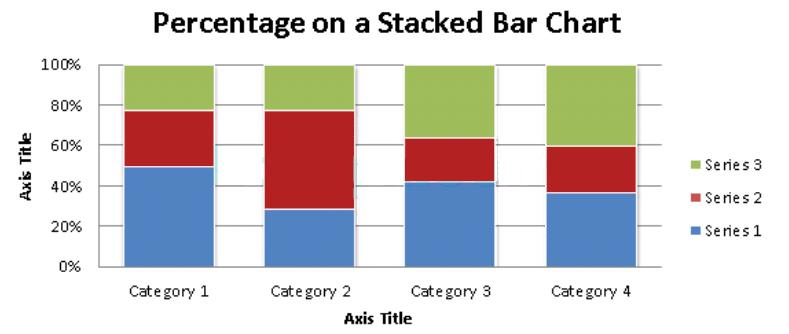Try yourself:The following chart represents the number of students of AMS careers at its Lucknow center who passed either the CAT exam or XAT exam or CET exam or none of these exams. Assume that there are no students who passed more than one exam.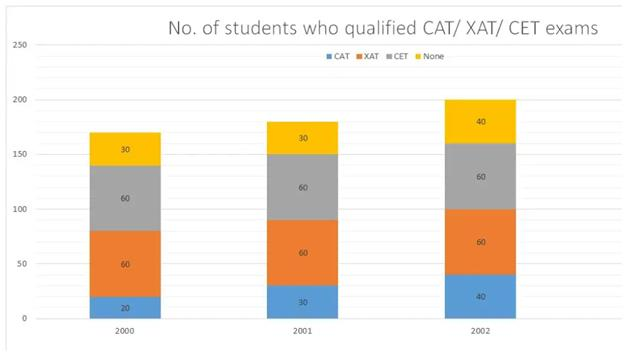What was the percentage of students who cleared CAT in 2000?

Solved Examples

Q.1. Answer the question based on the chart below.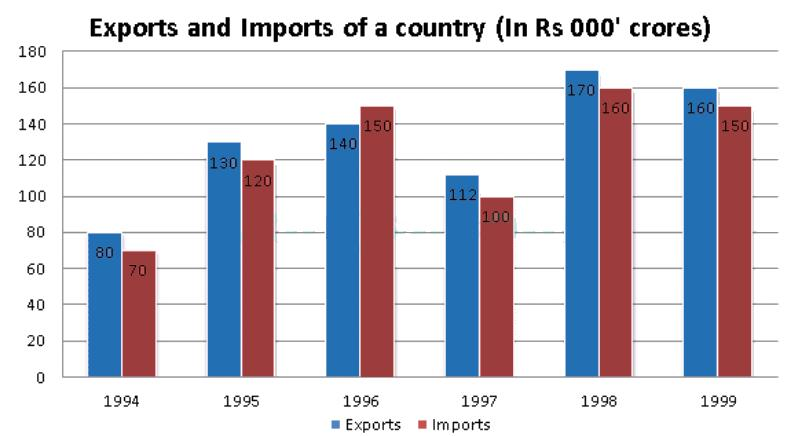(i) In how many of the given years was the exports at least 10% more than the imports?

(A) 0       (B) 1       (C) 2        (D) 3       (E) 4

Solution:

(a) In 1994, exports = 80

Imports = 70

10% of imports = (70 x (10/100) = 7)

⇒, Exports > imports + 10% of imports

(b) In 1995, exports = 130

Imports = 120

10% of Imports =(120 x (10/100) = 12)

⇒ Exports < imports + 10% of imports

(c) In 1996, exports = 140

Imports =  150

⇒ Exports < Imports so we need not consider this case.

(d) In 1997, Exports = 112

Imports = 100

10% of Imports = (100 x (10/100) = 10)

⇒ Exports > Imports + 10% of imports(e). In 1998, Exports = 170

Imports = 160

10% of Imports = (160 x (10/100) = 16)

⇒ Exports < Imports + 10% of Imports

(f) Exports =  160

Imports = 150

10% of Imports = (150 x (10/100) = 15)

⇒ Exports < Imports + 10% if Imports

⇒The given condition was satisfied in two years.

Choice (C) is the correct answer.

(ii) What were the average exports for the given period (in Rs. 000' crores)?

(A) 145       (B) 132       (C) 126       (D) 119       (E) 138

#### Solution: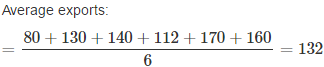Thus choice (B) is the right answer.

(iii) From 1995 to 1999, in which year was the percentage growth In exports, when compared to the previous year, the highest?

(A) 1995       (B) 1996       (C) 1997       (D) 1998       (E) 1999

Solution: Export in a year exceeded that in the previous year in 1995, I996 and I998. Percentages by which exports in I995, I996 and 1998 exceed the exports in the previous year were: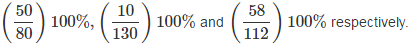Only in I995 was the growth more than 60%

Thus choice (A) is the correct answer.

(iv) What is the simple average annual growth rate in the imports from I994 to 1999?

(A) 15      (B) 18      (C) 19      (D) 21      (E) 23

Solution:

Imports in 1994 (in '000 crores) = 70

Imports in 1999 (in '000 crores) = 150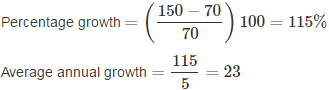Thus choice (E) is the correct answer.

(v) Among the years in which the imports, as well as exports, exceed those in the previous years, In how many years was the percentage increase in imports less than the percentage increase in exports?

(A) 0       (B) 1       (C) 2       (D) 3       (E) 4

Solution: The imports, as well as exports, exceeded those in the previous years in I995, I996 and 1998. In none of the years was the given condition satisfied.

Thus choice (A) is the correct answer.

Q.2. Production of paper (in lakh tonnes) by three companies X, Y and Z over the years. Study the graph and answer the questions that follow.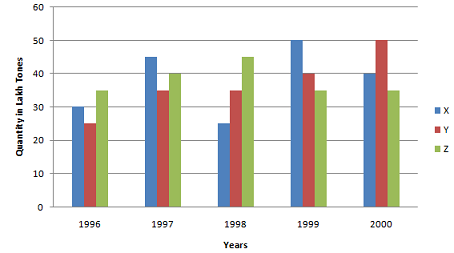(i) What is the difference between the production of company Z in 1998 and company Y in 1996?

Ans: Required difference

= [(45 - 25) * 1,00,000] tones

= 20,00,000 tons.

(ii) What is the ratio of the average production of company X in the period 1998-2000 to the average production of company Y in the same period?

Ans:  Average production of company X in the period 1998-2000 = [1/3 * (25 + 50 + 40)] = (115/3) lakh tons. Average production of company Y in the period 1998-2000 = [1/3 * (35 + 40 + 50)] = (125/3) lakh tons. Required ratio = (115/3)/(125/3) = 115/125 = 23/25

(iii) What is the percentage increase in the production of company Y from 1996 to 1999?

Ans: Percentage increase in the production of company Y from 1996 to 1999 = [(40 - 25)/25 * 100]% = (15/25 * 100)% = 60%

(iv) The average production for five years was maximum for which company?

Ans: For company

• X = [1/5 * (30 + 45 + 25 + 50 + 40)] = 190/5 = 38
• For company Y = [1/5 * (25 + 35 + 35 + 50 + 40)] = 185/5 = 37
• For company Z = [1/5 * (35 + 40 + 45 + 35 + 35)] = 190/5 = 38
• Average production of five years in maximum for both the companies X and Z.

(v) In which year was the percentage of production of company Z to the production of company Y the maximum?

Ans: The percentage of production of company Z to the production of company Z for various years are:

For 1996 = (35/25 * 100)% = 140%
For 1997 = (40/35 * 100)% = 114.29%
For 1998 = (45/35 * 100)% = 128.57%
For 1999 = (35/40 * 100)% = 87.5%
For 2000 = (35/50 * 100)% = 70%

Clearly, this percentage is highest for 1996.

The document Bar Graphs - Introduction and Examples (with Solutions), Data Interpretation LR Notes | EduRev is a part of the Banking Exams Course General Aptitude for GATE.
All you need of Banking Exams at this link: Banking ExamsUse Code STAYHOME200 and get INR 200 additional OFF Use Coupon Code
All Tests, Videos & Notes of Banking Exams: Banking Exams

### Top Courses for Banking Exams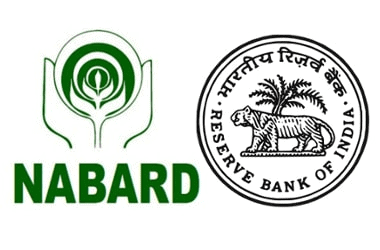## General Aptitude for GATE

61 videos|67 docs|88 tests

### Top Courses for Banking ExamsTrack your progress, build streaks, highlight & save important lessons and more!

,

,

,

,

,

,

,

,

,

,

,

,

,

,

,

,

,

,

,

,

,

,

,

,

;